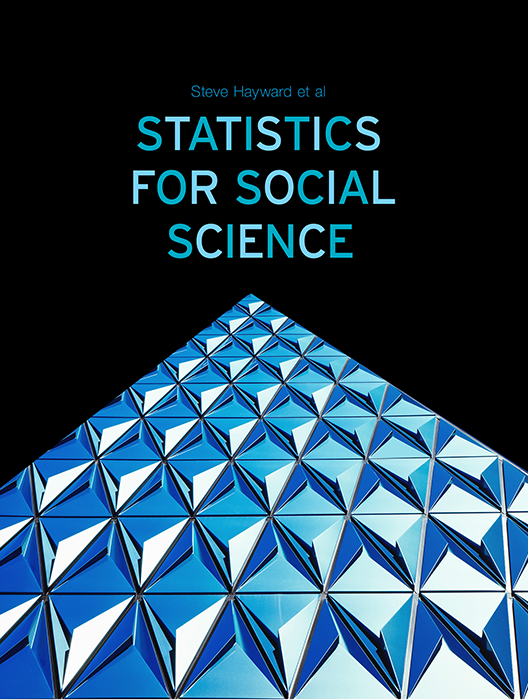# Statistics for Social Science

Lead Author(s): Stephen Hayward

Student Price: Contact us to learn more

Statistics for Social Science takes a fresh approach to the introductory class. With learning check questions, embedded videos and interactive simulations, students engage in active learning as they read. An emphasis on real-world and academic applications help ground the concepts presented. Designed for students taking an introductory statistics course in psychology, sociology or any other social science discipline.

This content has been used by 8,525 students

## What is a Top Hat Textbook?

Top Hat has reimagined the textbook – one that is designed to improve student readership through interactivity, is updated by a community of collaborating professors with the newest information, and accessed online from anywhere, at anytime.

• Top Hat Textbooks are built full of embedded videos, interactive timelines, charts, graphs, and video lessons from the authors themselves
• High-quality and affordable, at a significant fraction in cost vs traditional publisher textbooks

## Key features in this textbookOur Statistics for Social Science textbook allows students to manipulate data, visualize the effects discussed, and explore Lightboard videos that feature instructor explanations to reinforce concepts and calculations.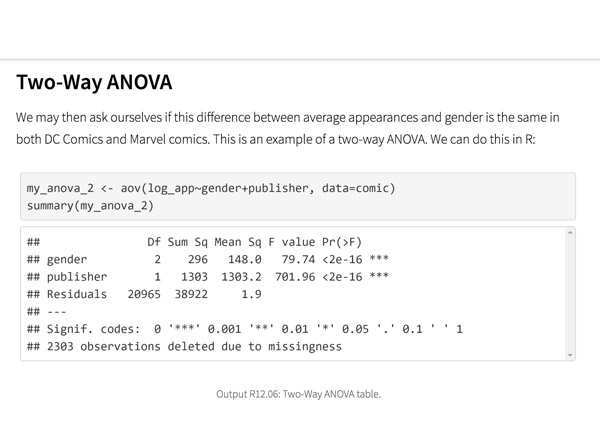Top Hat’s interactive offering includes a complementary module on using R software for data management, graphics, and conducting statistical analyses with examples and practice questions.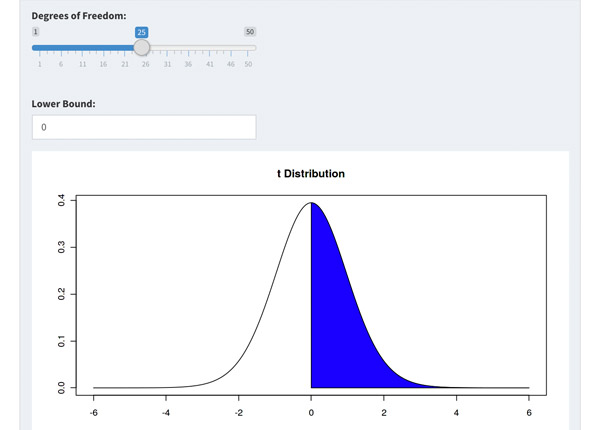Built-in assessment questions embedded throughout chapters so students can read a little, do a little, and test themselves to see what they know!

## Comparison of Social Sciences Textbooks

Consider adding Top Hat’s Statistics for Social Sciences textbook to your upcoming course. We’ve put together a textbook comparison to make it easy for you in your upcoming evaluation.

### Top Hat

Steve Hayward et al., Statistics for Social Sciences, Only one edition needed

### Pearson

Agresti, Statistical Methods for the Social Sciences, 5th Edition

### Cengage

Gravetter et al., Essentials of Statistics for The Behavioral Sciences, 9th Edition

### Sage

Gregory Privitera, Essentials Statistics for the Behavioral Sciences, 2nd Edition

### Pricing

Average price of textbook across most common format

#### Up to40-60%more affordable

Lifetime access on any device

#### $200.83 Hardcover print text only ####$239.95

Hardcover print text only

#### $92 Hardcover print text only ### Always up-to-date content, constantly revised by community of professors Content meets standard for Introduction to Anatomy & Physiology course, and is updated with the latest content ### In-Book Interactivity Includes embedded multi-media files and integrated software to enhance visual presentation of concepts directly in textbook Only available with supplementary resources at additional cost Only available with supplementary resources at additional cost Only available with supplementary resources at additional cost ### Customizable Ability to revise, adjust and adapt content to meet needs of course and instructor ### All-in-one Platform Access to additional questions, test banks, and slides available within one platform ## Pricing Average price of textbook across most common format ### Top Hat Steve Hayward et al., Statistics for Social Sciences, Only one edition needed #### Up to40-60%more affordable Lifetime access on any device ### Pearson Agresti, Statistical Methods for the Social Sciences, 5th Edition ####$200.83

Hardcover print text only

### Pearson

Gravetter et al., Essentials of Statistics for The Behavioral Sciences, 9th Edition

#### $239.95 Hardcover print text only ### Sage McConnell, Brue, Flynn, Principles of Microeconomics, 7th Edition ####$92

Hardcover print text only

## Always up-to-date content, constantly revised by community of professors

Constantly revised and updated by a community of professors with the latest content

### Top Hat

Steve Hayward et al., Statistics for Social Sciences, Only one edition needed

### Pearson

Agresti, Statistical Methods for the Social Sciences, 5th Edition

### Pearson

Gravetter et al., Essentials of Statistics for The Behavioral Sciences, 9th Edition

### Sage

Gregory Privitera, Essentials Statistics for the Behavioral Sciences, 2nd Edition

## In-book Interactivity

Includes embedded multi-media files and integrated software to enhance visual presentation of concepts directly in textbook

### Top Hat

Steve Hayward et al., Statistics for Social Sciences, Only one edition needed

### Pearson

Agresti, Statistical Methods for the Social Sciences, 5th Edition

### Pearson

Gravetter et al., Essentials of Statistics for The Behavioral Sciences, 9th Edition

### Sage

Gregory Privitera, Essentials Statistics for the Behavioral Sciences, 2nd Edition

## Customizable

Ability to revise, adjust and adapt content to meet needs of course and instructor

### Top Hat

Steve Hayward et al., Statistics for Social Sciences, Only one edition needed

### Pearson

Agresti, Statistical Methods for the Social Sciences, 5th Edition

### Pearson

Gravetter et al., Essentials of Statistics for The Behavioral Sciences, 9th Edition

### Sage

Gregory Privitera, Essentials Statistics for the Behavioral Sciences, 2nd Edition

## All-in-one Platform

Access to additional questions, test banks, and slides available within one platform

### Top Hat

Steve Hayward et al., Statistics for Social Sciences, Only one edition needed

### Pearson

Agresti, Statistical Methods for the Social Sciences, 5th Edition

### Pearson

Gravetter et al., Essentials of Statistics for The Behavioral Sciences, 9th Edition

### Sage

Gregory Privitera, Essentials Statistics for the Behavioral Sciences, 2nd Edition

## About this textbook

### Lead Authors

#### Steve HaywardRio Salado College

A lifelong learner, Steve focused on statistics and research methodology during his graduate training at the University of New Mexico. He later founded and served as CEO of Center for Performance Technology, providing instructional design and training development support to larger client organizations throughout the United States. Steve is presently lead faculty member for statistics at Rio Salado College in Tempe, Arizona.

#### Joseph F. Crivello, PhDUniversity of Connecticut

Joseph Crivello has taught Anatomy & Physiology for over 34 years, and is currently a Teaching Fellow and Premedical Advisor of the HMMI/Hemsley Summer Teaching Institute.

## Explore this textbook

Read the fully unlocked textbook below, and if you’re interested in learning more, get in touch to see how you can use this textbook in your course today.

# The Normal Distribution and Normal CurveA visualization of varying light and abstract curves.​ 

## Chapter Objectives

After you complete this chapter you will be able to:

•  Define a normal distribution in terms of its characteristics
•  Use the empirical rule to locate data values in a distribution
•  Use Chebyshev’s theorem to estimate the spread of a distribution
•  Determine whether a set of data are normally distributed
•  Calculate and interpret z-scores
•  Use z-scores to make comparisons across distributions

## Distributions of Data

As we saw in a previous chapter, distributions of data can be described and classified in any number of ways. The shape of graphed distributions tells us a lot about the spread of the data, i.e., how it is dispersed and how, or if, it tends to “clump up” around some data points. Here are some examples of data distributions and shapes: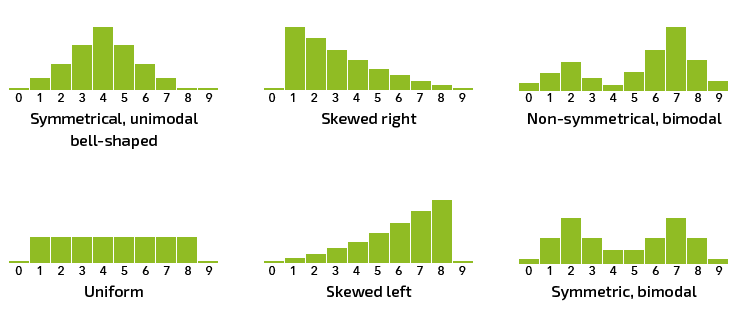Figure 3.1: Distribution shapes​

## The Normal Distribution

Most distributions of data in nature are said to be “normally distributed,” meaning that when graphed they tend to be unimodal and symmetrical and appear as a bell-shaped distribution, like the example at the upper left, above.

In a normal distribution, most scores are clustered around the middle of the distribution, with fewer scores out towards the “tails.” This also indicates the relative probability of selecting a given score by chance (i.e., if we were to draw a score at random from the distribution, we would be more likely to get a score nearer to the middle, or mean, than out towards the tails, where there are fewer scores). You can see this in the histogram below:Figure 3.2: Normal distribution histogram​

The scores we get from the populations we are interested in comparing will generally be considered to be normally distributed. This assumption provides the basis for the statistical tests we will use to make these comparisons.

Example: Housefly Wing Lengths

The chart below summarizes data on housefly wing lengths, providing a near-perfect example of how naturally occurring data tend to be normally distributed.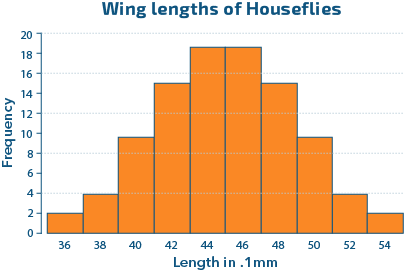Figure 3.3: Wing Lengths of HousefliesData Source: Sokal, R.R. and F.J. Rohlf, 1968. Biometry, Freeman Publishing Co., p 109. Original data from Sokal, R.R. and P.E. Hunter. 1955. A morphometric analysis of DDT-resistant and non-resistant housefly strains Ann. Entomol. Soc. Amer. 48: 499-507.​​

3.01

Most distributions in nature tend to be ________ distributed.

3.02

If you were to randomly select a score (data point) from a normally distributed data set, it would be most likely to be close to the ________.

3.03

If you were to randomly select a score (data point) from a normally distributed data set, it would be least likely to be close to the ________.

## The Normal Curve

The normal curve is a graphical representation of the normal distribution. It is sometimes seen “layered” over a histogram of data, as below: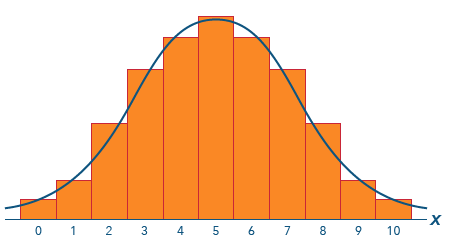Figure 3.4: Normal curve with histogram​

The familiar bell curve is used to represent the normal distribution, as it graphically depicts the way scores or values are dispersed or distributed. We often speak of the “area under the normal curve” because the area of any given portion of the curve corresponds to the proportion of scores in that portion.

## Characteristics of the Normal Distribution and Its Graph, the Normal Curve

Below are some important characteristics to keep in mind as we begin our study of the normal distribution and normal curve.

• The normal curve is bell-shaped and symmetric about the mean. Since more scores are near the center of the distribution, close to the mean, and fewer scores are near the tails, the area is greatest near the center.

You can see in the image below how data tend to cluster around the middle of the distribution. The mean of this distribution would be in the center of the middle portion, with the majority of scores lying closer to that mean, while fewer scores would lie out towards the tails.Figure 3.5: Distribution of scores​
• The mean, median and mode are equal. The mean locates the center of the distribution. Since it is symmetrical, the median and mode exactly overlie the mean. In an asymmetrical or skewed distribution the median and mean tend to be pulled towards one tail or the other in the direction of the skew.

Contrast the examples below. Notice how the mean, median and mode are identical in the symmetrical normally shaped distribution in the middle, but the mean and median are pulled to one side of the mode in the skewed distributions. This effect may be due to outliers in the data that are responsible for the skew. Outliers are extreme values in a distribution that have the effect of skewing the distribution. In extreme cases, the outlier(s) may severely distort the shape of the distribution. In such cases, the median may be more useful as a measure of central tendency than the mean. See the images just below for an example of the effect.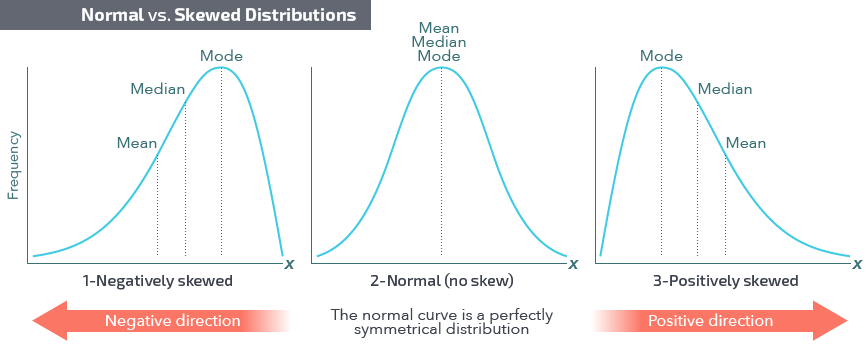Figure 3.6: Normal vs. skewed distributions​
• The probability associated with a range of values (scores) in the distribution is equal to the corresponding area under the curve. The area to the left or right of the mean corresponds to a probability of ½, or .5.

The shaded area of the curve below accounts for one-half of the scores in the distribution. It follows that if we were to randomly select a single score from the distribution, the probability of selecting a score from the shaded region would be equal to ½, or .5.Figure 3.7: Probability associated with one-half the area​

The total area under the normal curve is equal to 1. If you were to sum the probabilities of every score in the distribution they would sum to 1.

As redundant as this may seem, it is nevertheless important to keep in mind that since all scores in a distribution are included in the distribution’s graph, the sum of the probabilities associated with the individual scores must be equal to 1. This simple fact will play an important role in statistical tests to be introduced in coming chapters.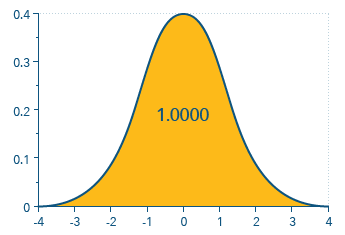Figure 3.8: Probability associated with the total area​
• The normal curve approaches, but never touches, the x-axis of the graph. There is always a probability associated with any possible score, no matter how slight. Note that this is true in theory, although many (or most) examples of graphs here and elsewhere appear to have the curve connecting to the x-axis. Please bear with us on this minor discrepancy!

This can be seen in the graph below, emphasizing how the distribution is in fact continuous beyond the limits shown for the graph.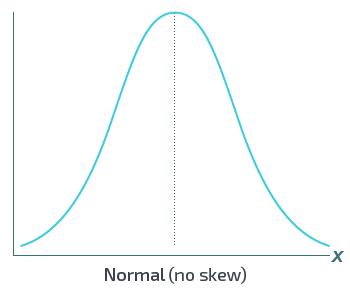Figure 3.9: Normal curve in relation to the x-axis​
• A normal distribution is defined by its mean and standard deviation, which also determine the shape of the graphed normal curve representing the distribution. The mean locates the center and the standard deviation indicates the variability and therefore how spread out the distribution is.
• The greater the standard deviation of the distribution, the greater the “spread” of the distribution’s graphed normal curve.

The image below illustrates how the graphed distributions vary as a function of their defining characteristics, the mean and standard deviation. Notice how the curves spread out in response to increases in the variance (which relates to the standard deviation, as you know).Figure 3.10: Spread of curves in relation to variance​​This Content is Locked
Only a limited preview of this text is available. You'll need to sign up to Top Hat, and be a verified professor to have full access to view and teach with the content.

3.04

The mean of a distribution of scores is determined to be to the right of (higher than) the median. What does that suggest about the distribution?

A

It is bimodal.

B

It has a negative skew.

C

More scores are above the mean than below.

D

It is positively skewed.

3.05

Two distributions, A and B, have the same mean, but Distribution A has σ = 2.0 and Distribution B has σ = 4.0. Which distribution has the greatest spread?

A

Distribution A has the greatest spread.

B

Distribution B has the greatest spread.

C

Both have the same spread.

D

Cannot be determined without a graph.

3.06

In a normal distribution, the sum of the probabilities of all scores below the mean is equal to ______.

A

1

B

.33

C

.50

D

.64

3.07

We are told that the mean, median and mode of a distribution of scores are not the same value. What else do we know about that distribution?

A

It is positively skewed.

B

The data are not normally distributed.

C

It is negatively skewed.

D

Cannot say without more information.

## The Empirical Rule

If data are normally distributed, knowing its mean and standard deviation enables us to determine the proportion of data that lies within any given range of the distribution. You will recall that the standard deviation serves as a “locator” for scores within the distribution. Since the mean and standard deviation determine the shape of the distribution, knowing those values allows us to determine the proportion of scores within any given portion of that distribution.

The empirical rule states that, for data with a symmetric, bell-shaped distribution like the normal curve shown below, the normal curve area has the following characteristics:

•  About 68% of the scores lie within one standard deviation of the mean.
• About 95% of the scores lie within two standard deviations of the mean.
• About 99.7% of the scores lie within three standard deviations of the mean.This Content is Locked
Only a limited preview of this text is available. You'll need to sign up to Top Hat, and be a verified professor to have full access to view and teach with the content.

Probability and the Empirical Rule

Since 68% of the distribution’s scores lie within one standard deviation of the mean, that also tells us the probability of selecting a score at random that lies within one standard deviation of the mean is approximately .68, or 68%. Likewise, the probability of selecting a score that lies within two standard deviations of the mean is approximately .95, or 95%.

This will be important to know when we begin making statistical decisions based on information from sample data.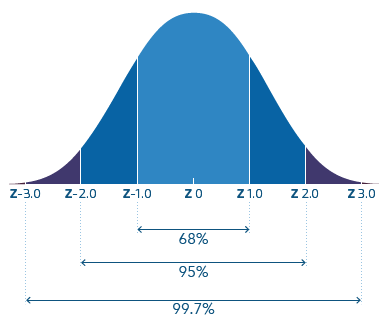Figure 3.11: The Empirical rule and standard deviations​

Example: Probability of a Score

Using the normal curve graph above, determine the probability of randomly selecting a score from the distribution that falls between 1 and 2 standard deviations above the mean.

Solution

• 95% of scores lie within 2 standard deviations of the mean, above and below.
• 68% of scores lie within 1 standard deviation of the mean, above and below.
• We want scores above the mean, so need to calculate ½ the difference between 95% and 68%.
• 0.5 (95% - 68%) = 0.5 (0.27) = 13.5% of scores lie between 1 and 2 standard deviations above the mean.

3.08

Assuming a normal distribution of data, what is the probability of randomly selecting a score that is more than 2 standard deviations below the mean?

A

.05

B

.025

C

.50

D

.25

3.09

Assuming a normal distribution of data, what proportion of scores would be found between 1 and 3 standard deviations above the mean?

A

34%

B

5%

C

15.85%

D

27%

3.10

The probability of selecting a score that lies within two standard deviations of the mean is approximately:

A

99%

B

95%

C

68%

D

32%

## Determine Whether a Data Set is Normally Distributed

One of the ways we can use the empirical rule is to determine whether a sample of data comes from a normally distributed population. If the proportions of the data are close to expected proportions for a normal distribution and a histogram (or stem-and-leaf plot or dot plot, etc.) shows a unimodal and approximately symmetrical distribution of scores, it is very likely that the sample came from a normal distribution.

The steps to follow are summarized in the table below.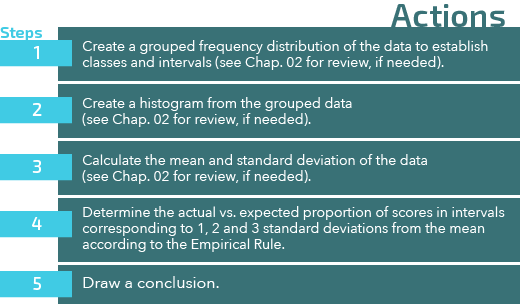Figure 3.12: Determine normalcy of distribution​

Below is an example of how you can use this information to determine whether a sample of data is likely to have come from a population that is normally distributed.

Example: Critical Thinking Scores

Here is a hypothetical set of data representing scores on a measure of critical thinking ability from a sample of 60 people. The data in raw form don’t really tell us much, nor can we do much with them without first organizing and summarizing them in some way. We might first want to know if it makes sense to assume they come from a normally distributed population, as that will, in turn, affect how we might go about analyzing the data.

Critical Thinking Scores for 60 Participants

80,81,84,91,92,95,96,98,101,102,103,104,104,104,106,106,107,108,108,109,109,110,111,111,111,112,112,113,114,114,115,115,115,116,116,117,118,119,119,120,121,122,122,123,124,124,125,126,128,128,129,132,133,134,134,135,138,139,143,144

Step 1: Create a grouped frequency distribution of the data to establish classes and frequencies.Figure 3.13: Critical thinking scores​

Step 2: Create a histogram from the grouped data.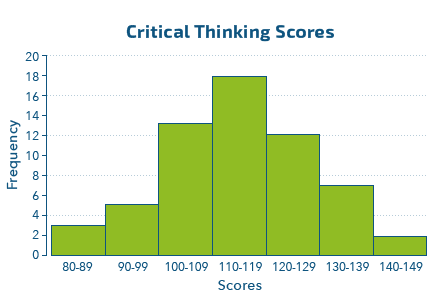Figure 3.14: Critical thinking scores histogram​

Step 3: Calculate the mean and standard deviation of the data.

Step 4: Determine the actual vs. expected proportion of scores in intervals.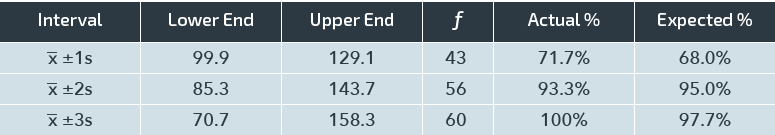Figure 3.15: Actual vs. expected proportions​

Step 5: Draw a conclusion.

Given the outcome of the 68-95-99.7 proportions test and the observation that the histogram shows the data are unimodal with an approximately symmetric distribution, we can conclude that the scores in this sample come from a population that is normally distributed. The distribution has a mean of approximately 115 and a standard deviation of approximately 15.

## Determine Mean and Standard Deviation of a Known Distribution

If we should be given information about a distribution of scores that does not include the raw scores or mean and standard deviation, we may be able to use the empirical rule to calculate those from the data provided. If we know that a certain range of scores accounts for a given percentage of the data, we can use that information to make determinations about the center and spread of the data as long as the data can be assumed to be normally distributed. This can probably best be seen in an example.

Example: Find Mean and Standard Deviation of a Distribution

We are told that 95% of individuals taking the Wechsler Adult Intelligence Scale (WAIS) score between 70 and 130. IQ scores are well researched and generally are normally distributed, so we can assume the data here are likewise.

We know from the empirical rule that 95% of scores lie within two standard deviations of the mean, either above or below, so 95% covers a total of four standard deviations.

3.11

Given a normal distribution of scores with µ = 85 and σ = 17.5, what raw score lies at the upper boundary of the interval that includes scores within one standard deviation of the mean?

3.12

For the distribution given in question 3.11 above, what percentage of scores would lie in the interval bounded by raw scores of 67.5 and 85?

3.13

Given a normal distribution of scores with 95% of the scores centered about the mean between the scores of 32 and 48, what is the variance?

## Chebyshev’s Theorem

Chebyshev’s theorem applies to any distribution, whether or not it is bell-shaped, and provides a way to estimate the spread of a distribution without regard to whether the data are normally distributed. The theorem gives us a way to determine the minimum percent of data values that fall within a given number of standard deviations from the mean. The value of the theorem is that it enables one to determine that at least a certain percentage of scores can be expected to fall within a given range.

To use the theorem, let k represent the number of standard deviations in the range. Note that k must be greater than 1. For k > 1, the proportion of any data set within k standard deviations of the mean is at least 1 – (1 / k2). The relationship can be expressed as:

• k = 2: in any data set, at least 1 – ¼ = ¾, or 75%, of the data lie within 2 standard deviations of the mean:
•  k = 3: in any data set, at least 1 – 1/32 = 8/9, or 88.9%, of the data lie within 3 standard deviations of the mean: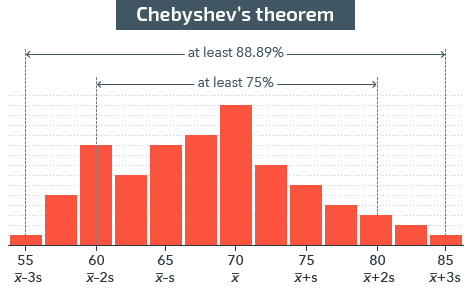Figure 3.16: Chebyshev’s theorem​

Example: Using Chebyshev’s Theorem

A graduate student using laboratory rats as subjects in an animal learning lab experiment has collected data for his thesis project on the average number of trials needed to extinguish a previously learned sequence of behaviors leading to a food pellet reward. He has determined that the mean number of trials needed to extinguish the behavior is 84 with a standard deviation of 16.0. The data do not appear to be normally distributed. As part of his discussion for the thesis, the student wants to report the minimum proportion of rats requiring between 60 and 108 trials to extinguish the behavior sequence. His solution is as follows:

Step 1: The first step is to determine a value for k, where k = the number of standard deviations the score is from the mean, which in turn equals the distance between the mean and either of the limits, i.e., 60 or 108, divided by σ.

Step 2: To determine the proportion of scores within 1.5 standard deviations of the mean (one score per subject), use the formula for Chebyshev’s theorem:

Step 3: At least 56% of the subjects require from 60 to 108 trials to extinguish the behavior sequence.

3.14

Click on the area that includes at least 75% of the scores in the distribution.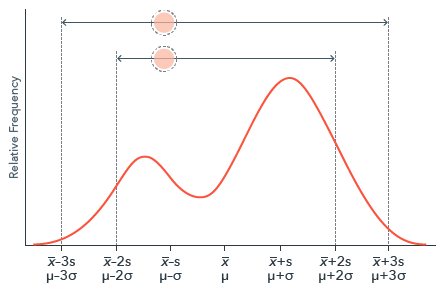## Introduction to z-Scores

A z-score is based on raw scores and standard deviations and enables comparisons to be made across distributions. It gives the distance in standard deviations from a raw score to the mean of the distribution.

While a standard deviation only relates to the specific distribution it came from, a z-score is independent of the distribution and for that reason is used to make comparisons across distributions. A z-score is often referred to as a standard score for this reason. There is more about this important feature of z-scores below, but first, we’ll take a look at the underlying logic of z-scores.

### The Logic of z-Scores

Since a z-score represents the distance a raw score is from the mean of the distribution, calculating a z-score is simply a matter of dividing the difference between the raw score and the mean by the calculated standard deviation of the distribution. This tells you the number of standard deviations the score is away from the mean.

The distance from the mean, expressed in standard deviations, is the z-score associated with that raw score.

A negative z-score indicates the associated raw score is below the mean, and a positive z-score is associated with a raw score that is above the mean. For example, a z-score of -1.0 and a z-score of +1.0 are “located” in opposite directions relative to the mean but are exactly the same distance from the mean, since the distribution is symmetrical.

## The Standard Normal Distribution

There is an infinite number of normal distributions, each relating to a specific set of data and each with its own mean and standard deviation. The normal distribution with a mean of 0 and a standard deviation of 1 is called the standard normal distribution. The horizontal axis corresponds to z-scores since z-scores, in turn, equate to the number of standard deviations and can be used to locate individual scores in the same way standard deviations can be used to locate scores.Figure 3.17: The Standard normal distribution​

### Comparing z-Scores

Comparing the graph above to the previous graph showing standard deviations on the horizontal axis, you can see that a z-score cuts off the same area of the normal curve as the standard deviation of the same value. But since a z-score is “universal,” i.e., applies to any distribution, it can be used to compare scores across distributions, which is why z-scores are referred to as “standard scores” and their graph as the “standard normal distribution.”

Example: Compare Scores in Two Distributions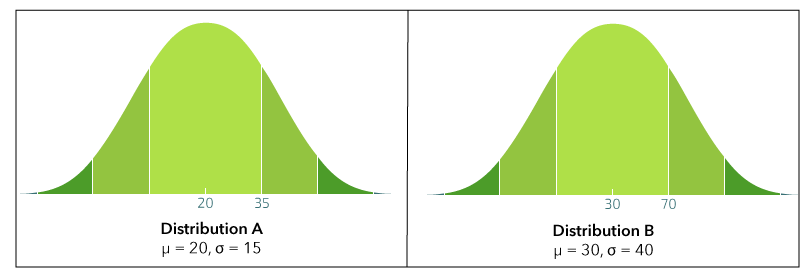Figure 3.18:​ Distribution Example A and Distribution Example B​

A score of 35 on Distribution A has a z-score of 1.0; a score of 70 on Distribution B also has a z-score of 1.0. Both are exactly one standard deviation above the mean. The raw scores are different but the z-scores are the same. A score of 35 on Distribution A is the same distance from the mean as a score of 70 on Distribution B. Both scores are at approximately the 84th percentile of their respective distributions of scores.

While a raw score of 70 might sound like a much higher score than a score of 35, we can see from this that, taken in the context of the distributions the scores are taken from, they really are very similar in terms of their relationship to the scores around them.

This can be important when comparing, for example, scores on performance tests or inventories of personality characteristics. The testing instruments may have different means and standard deviations, but by converting scores to z-scores we can still make comparisons between them.

3.15

Determine whether the following statement is true or false. If false, rewrite it as a correct statement: It is impossible to have a z-score of 0.

A

False. A $z$-score of 0 can occur 68% of the time

B

False. A $z$-score of 0 is a standardized score that is equal to the standard deviation

C

True

D

False. A $z$-score of 0 is a standardized score that is equal to the mean

3.16

The mean for a math test is 65 and the standard deviation is 8.0. The mean for a history test is 32 and the standard deviation is 3.0. A student who took both tests scored 71 on the math test and 36 on the history test. On which test did the student have a better score?

3.17

Based on what you know about the empirical rule and z-scores, and assuming a normal distribution, what is the approximate percentile rank of a score that is associated with a z-score of 0?

## Calculation of z-Scores

The math involved in figuring z-scores is simple subtraction and division—nothing fancy or complicated, and very straightforward. They are calculated using the mean and standard deviation of the distribution the raw score is included in.

In its simplest form, the basic formula for a z-score is:

Note: It is important to subtract the mean of the distribution from the raw score and not the other way around. This ensures that a raw score that is below the mean is assigned a z-score that is negative, and a raw score that is above the mean is assigned a z-score that is positive.

Example 1: Calculate a z-Score

An example distribution we used earlier had a mean of 4.5 and a standard deviation of 1.1.

In that distribution, a raw score of 5 would have a z-score calculated as

The raw score of 5 is located exactly 0.45 (45/100) of one standard deviation above the mean.

Example 2: Calculate a z-Score

Scores for a group of 30 people on a personality subtest scale were determined to be:

101,102,103,104,104,104,106,106,107,108,108,109,109,110,111,111,111,112,112,113,114,114,115,115,115,116,116,117,118,119

The test administrator needs the z-score of a person who scored 115 on the test. To determine this, the administrator worked through the following steps:

Step 1: Used technology to calculate the mean and standard deviation as µ = 110.3, σ = 5.1.

Step 2: The z-score associated with a raw score of 115 was then calculated as

3.18

A student union cafeteria worker checked the weight of ten half-pound bags of whole bean coffee and recorded the following weights in pounds: 0.48, 0.51, 0.47, 0.49, 0.49, 0.50, 0.52, 0.48, 0.49, 0.51.

What is the mean weight of this group of coffee bags?

3.19

A student union cafeteria worker checked the weight of ten half-pound bags of whole bean coffee and recorded the following weights in pounds: 0.48, 0.51, 0.47, 0.49, 0.49, 0.50, 0.52, 0.48, 0.49, 0.51.

What is the standard deviation of the weight of these coffee bags?

3.20

A student union cafeteria worker checked the weight of ten half-pound bags of whole bean coffee and recorded the following weights in pounds: 0.48, 0.51, 0.47, 0.49, 0.49, 0.50, 0.52, 0.48, 0.49, 0.51.

What is the z-score associated with the bag weighing 0.50 lbs.?

There is an online z-score calculator here. Online calculators such as this may be useful for experimenting to see how outcomes vary as a result of the data you can input, and for checking results on the fly.

### Finding a Raw Score Given a z-Score

Another way we can use the z-score formula is to find the raw score that is (or would be) associated with a particular z-score. This could be useful in a situation where you have a distribution of scores and need to determine what raw score would correspond to a given z-score.

The formula for finding a raw score can be derived from the z-score formula as:

### Logic

If the mean and standard deviation are known, the z-score represents the distance from the mean to the raw score. Multiplying the z-score by the standard deviation tells us how far the raw score is from the mean in terms of the original data values. Adding that to the mean gives the exact location, or score. (If the z-score is negative, adding the negative distance ends up being a subtraction.)

Example: Convert a z-Score to a Raw Score

Determine the raw score (weight) of the coffee bag in the question set above that has a z-score of 1.65.

## The Empirical Rule Applied to z-Scores

Since z-scores represent the distance a score is from the distribution’s mean, the empirical rule can be used here as well. It tells us that approximately 68% of the scores in a normal distribution lie within one standard deviation above or below the mean. Since one standard deviation corresponds to a z-score of ± 1.0, it follows that 68% of the distribution’s scores will have a z-score between -1.0 and +1.0. In like fashion, approximately 95% of a distribution’s scores will have z-scores between -2.0 and +2.0, and approximately 99.7% of a distribution’s scores will have z-scores between -3.0 and +3.0.

Recall that the mean, by definition, has a z-score of zero, so the positive and negative z-scores balance around that point.

### Probability

Since 68% of the distribution’s scores have z-scores between -1.0 and +1.0, that also tells us the probability of selecting a score at random that has a z-score between -1.0 and +1.0 is approximately .68, or 68%. Likewise, the probability of selecting a score that has a z-score between -2.0 and +2.0 is approximately .95, or 95%.

3.21

Below is a graph of a distribution of SAT scores with µ = 500 and σ = 100. Click on an area of the graph that includes approximately 34% of the scores of the distribution.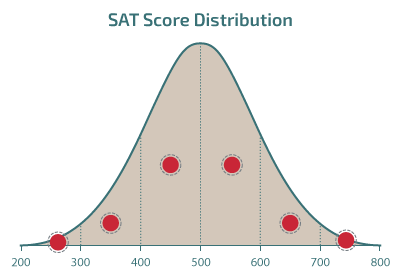## The Normal Curve and Probability

We can start to take a look at probabilities associated with groups of scores by first looking at “slices” of the normal curve and the probabilities associated with those.

The graph below provides a more detailed breakdown of the exact proportions of data included in slices of the normal curve. The exact proportions vary slightly from the approximations we’ve previously seen expressed as “68-95-99.7.”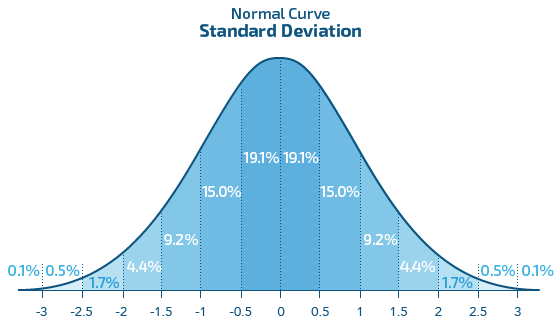Figure 3.19: Normal Curve and Probability​

We know that areas under the normal curve correspond to the proportion of data, or scores, included in those areas and, therefore, the probability associated with scores in those areas. From the graphic above, we can determine that, for example, 19.1% of scores will fall between the mean and ½ standard deviation either above or below the mean, 15% will fall between 0.5 and 1.0 standard deviations, and so forth. More importantly, that also gives us the proportion of z-scores in those intervals, thus enabling us to determine with some accuracy the probabilities associated with various ranges of z-scores for a normally distributed set of data.

Note: In another chapter, you’ll learn an even more exact method of determining probabilities associated with individual scores, so consider this the first step in that direction.

Example: Probabilities Associated with z-Scores

From the graph above, we can see, for example, the probability of selecting a score with a z-score between 0 and 0.50 from a set of normally distributed data is .191, or 19.1%. In like fashion, the probability of selecting a score with a z-score between -1.5 and -1.0 is .092, or 9.2%.

3.22

Given a large data set, what is the probability of a z-score starting with -1?

3.23

Given a large data set, what is the probability of randomly selecting a score between 1 and 2 standard deviations below the mean?

### The Normal Curve and Percentiles

We can also use the normal curve areas to approximate percentiles. You’ll recall that percentiles divide a data set into hundredths.

The 50th percentile, or Q2, is the score that lies at the midpoint of the distribution and cuts off the lower 50% of the scores in that distribution. In any distribution, the median is equal to the 50th percentile. Since the mean and median are the same in a normal distribution, it follows that for a normally distributed set of data, mean = median = 50th percentile.

Referring to the graph above showing slices of areas under the normal curve, we can also see that a score with a z-score of 0.5 (½ standard deviation from the mean) would be at approximately the 69th percentile (50% + 19.1%).

## Case Study: U.S. Household Incomes

The Economic Education and Outreach division of the Federal Reserve Bank of San Francisco hosts an educational site of teaching resources with statistics and discussions here. There are several series of slides that you can click through and then respond to questions. For convenience, the images presented in the first slide series, about U.S. Household Incomes, are reproduced below.

### Case Study Question 3.01

Case Study Question 3.01

Ignoring incomes that are equal to or greater than $200,000, what kind of shape does the distribution have? Click here to see the answer to Case Study Question 3.01. Cast Study Question 3.02 What percentage of U.S. households earned between$75,000 and $79,000 in 2014? A 0.5 B 1.2 C 2.8 D 6.6 Case Study Question 3.03 Enter in the difference between the median and mean average household income in 2014. (Enter in your answer as an absolute value with no commas) Case Study Question 3.04 50% of U.S. households earned up to$$\_\_\_\_\_\_$ in 2014. (Enter in your answer without commas)

### Case Study Question 3.05

Case Study Question 3.05

Why would household incomes over \$200,000 be grouped together?

Click here to see the answer to Case Study Question 3.05.

### References

U.S. Household Incomes: A Snapshot. (2015, October 5). Retrieved from Federal Reserve Bank of San Francisco: http://www.frbsf.org/education/teacher-resources/datapost/microeconomics/income-inequality-us-household-incomes

## Pre-Class Discussion Questions

### Class Discussion 3.01

Class Discussion 3.01

Describe a normal distribution in terms of its shape.

Click here to see the answer to Class Discussion 3.01.

### Class Discussion 3.02

Class Discussion 3.02

How does the normal curve relate to the normal distribution?

Click here to see the answer to Class Discussion 3.02.

### Class Discussion 3.03

Class Discussion 3.03

What is the importance of the Empirical Rule?

Click here to see the answer to Class Discussion 3.03.

### Class Discussion 3.04

Class Discussion 3.04

How does Chebyshev’s Theorem compare in function to the Empirical Rule?

Click here to see the answer to Class Discussion 3.04.

### Class Discussion 3.05

Class Discussion 3.05

What is the underlying logic of z-scores?

Click here to see the answer to Class Discussion 3.05.This Content is Locked
Only a limited preview of this text is available. You'll need to sign up to Top Hat, and be a verified professor to have full access to view and teach with the content.

## Answers to Case Study Questions

### Answer to Case Study Question 3.01

The shape is skewed right, suggesting that more people have incomes less than the average because the median is to the left of the mean. The mean is elevated because of the outliers with very high incomes.

Click here to return to Case Study Question 3.01.

### Answer to Case Study Question 3.05

They were grouped together because they include outliers that would otherwise extend the categories to such an extent it would hinder interpretation.

Click here to return to Case Study Question 3.05.

## Answers to Pre-Class Discussion Questions

### Answer to Class Discussion 3.01

Normal distributions are unimodal and symmetrical and appear as bell-shaped distributions when graphed.

Click here to return to Class Discussion 3.01.

### Answer to Class Discussion 3.02

The normal curve is a graph of the normal distribution.

Click here to return to Class Discussion 3.02.

### Answer to Class Discussion 3.03

The Empirical Rule summarizes the proportion of scores that lie within given ranges within the distribution.

Click here to return to Class Discussion 3.03.

### Answer to Class Discussion 3.04

Whereas the Empirical Rule applies only to normally distributed data sets, Chebyshev’s Theorem can be used to estimate the spread of a distribution without regard to whether the data are normally distributed.

Click here to return to Class Discussion 3.04.

### Answer to Class Discussion 3.05

A z-score is a standardized score representing the distance a given score lies from the mean of its distribution expressed in terms of standard deviations. A score with a corresponding z-score of 1.0 lies exactly one standard deviation away from the mean.

Click here to return to Class Discussion 3.05.

### Image Credits

 Image courtesy of littlevisuals.co in the Public Domain.